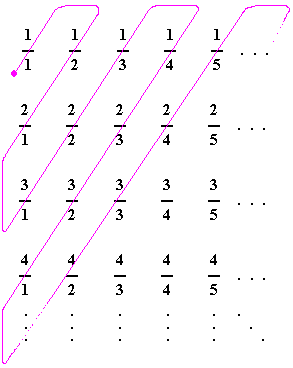# My school teacher didn't teach me this

Probability Level 1Which of the following statements is/are true?

$A)$ The set of all real numbers in the interval $(0,1)$ is not countable.

$B)$ The set of all rational numbers in the interval $[0,1]$ is not countable.

×